• The perceptron A probabilistic model for information storage and organization in the brain
• 本文提供的信息提供Perceptron设备的一般操作说明，工程数据和维护程序。 Perceptron对此处提供的信息或使用本信息不承担任何义务或责任，使用此类信息的风险由接收者自担。 对于因使用此信息而造成的任何直接，间接...
感知器信息通知
本文提供的信息提供Perceptron设备的一般操作说明，工程数据和维护程序。 Perceptron对此处提供的信息或使用本信息不承担任何义务或责任，使用此类信息的风险由接收者自担。
对于因使用此信息而造成的任何直接，间接，偶然或其他形式的损害，Perceptron概不负责，包括但不限于利润或收益的损失，使用权的损失或损害， 设备或其他财产损失或人员伤害。
Perceptron向非Perceptron人员提供，提供和传达此类信息，并不构成对此类信息的明示或暗示认可，促进或承担责任。 除服务手册或其他书面说明中明确规定的内容外，应警告接受者，请勿擅自维修Perceptron设备及相关活动，并且此类行为可能会严重损坏该设备，该设备发生故障， 人身伤害或财产损失。
如果未经Perceptron事先书面授权，由Perceptron人员以外的任何人对此类设备进行未经授权的维护，安装，服务，拆卸，调整和/或修理，则Perceptron对设备提供的保证将作废， 产品性能可能受到不利影响
Perceptron不做任何陈述或保证，不会进行任何改进，升级或更改。 为了技术进步，本手册中的信息如有更改，恕不另行通知。 本文档中的示例和图表仅用于说明目的，可能与系统上实际显示的示例和图表有所不同。
TriCam，TriLite，VeriStar，DDM，OCF，LASAR，Wheelworks，ScanWorks，IPNet和Perceptron是Perceptron，Inc.和DataCam，Micro-Flash，Visual Fixturing，Sensing the Future，Optiflex，Focus on Process， LinearStar和InterSystems是Perceptron，Inc.的商标。本文所述的硬件，软件和过程可能受到以下一项或多项专利的保护：US 4541721； US 4541721； US 4541721； US 4541721； US 4541721。 US 4645348； US 4647208； US 4666303； US 4745469； US 4841460； US 4862598； US 4899218； US 5006721； US 5078496，US75 / 728,106，EP 0543900； CA 1294427； CA 1304932； EP 280941； EP 306731； DE P3875984.5； DE P3877655.0； 案例＃4226-200037和案例＃4226-200038; 和6,180,939 B1。
在美利坚合众国印刷。 本出版物的任何部分均不得通过现在已知或以后发现的媒体以任何形式或通过任何方式复制，分发，存储在检索系统中或进行传播，包括但不限于印刷，电子，机械，影印，电子复印， 未经Perceptron，Inc.事先许可，以录音或其他方式进行录制，以及在网上公开显示和传输此作品的权利。本文档包含专有和机密技术信息。 本文档及其中的信息仅供分配其的客户和设施使用。 将该材料转移给未经授权的人员或企业实体，违反了Perceptron在美国版权法下的权利。
介绍
本文档包含使用Perceptron控制器时的通信规范。 该规范是一种严格的命令和响应通信类型，每个设备在通信方案中仅扮演一个角色，无论是主设备还是从设备，并且发出的每个命令都会收到强制性的肯定或否定确认。 下一节列出并记录了这些命令，而附录中列出了可用的通信协议的类型。
定时与同步
以下是遇到的典型配置的示例。 但是，它们不是唯一的。 有关确切的详细信息，请参阅项目的特定文档。
自动装配系统
在典型的Autofit Perceptron系统中，涉及三个或更多设备：Perceptron控制器，PLC和一个或多个机器人。 测量周期示例将遵循以下命令序列： PLC to Perceptron Robot to Perceptron
Set Color Set Part Id Start Cycle Get Routine Id WaitForFrame At Position Leaving Position End Cycle
固定量规
在典型的固定测量Perceptron系统中，涉及两个设备：Perceptron控制器和PLC。 测量周期示例将遵循以下命令序列：
PLC to Perceptron
Set Part Id Start Cycle Wait For All Data Acquired Wait For Cycle Complete Get Alarms End Cycle
分阶段测量
在典型的分阶段计量Perceptron系统中，涉及两个设备：Perceptron控制器和PLC。 测量周期示例将遵循以下命令序列：
PLC to Perceptron
Set Part Id Set Process Id Start Cycle Start Phase Wait For All Data Acquired Wait For Phase Complete Wait For Cycle Complete Get Alarms End Cycle
灵活的测量系统
在典型的灵活测量Perceptron系统中，涉及三个或更多设备：Perceptron控制器，PLC和一个或多个机器人。 在典型的FMS中，使用温度补偿，Perceptron系统可以指示要运行的例程。 PLC将使用“查询机器人补偿”命令来决定是否应运行补偿例程。 测量周期示例将遵循以下命令序列：
PLC to Perceptron Robot to Perceptron
Set Part Id Query Robot Compensation Start Cycle - Get Routine Id
                               Measure Position

Wait For Cycle Complete Get Alarms End Cycle
在某些系统中，PLC可以指示要运行的例程。 测量周期示例将遵循以下命令序列：
PLC to Perceptron Robot to Perceptron
Set Part Id Query Robot Compensation Start Cycle Set Routine Id Measure Position
Wait For Cycle Complete Get Alarms End Cycle
机器人制导系统
在典型的机器人引导Perceptron系统中，涉及三个或更多设备：Perceptron控制器，PLC和一个或多个机器人。 测量周期示例将遵循以下命令序列：
PLC to Perceptron Robot to Perceptron
Set Part Id Start Cycle Wait For Frame Wait For Cycle Complete End Cycle
指令
以下是Perceptron控制器支持的所有命令的字母顺序引用。 并非所有命令都适用于所有设备或项目。 请始终咨询您的特定项目文档和Perceptron代表，以澄清您可能遇到的任何问题。
Example Command (0x00)
以十六进制表示命令名称和命令编号。
Description
描述命令的目的。
Format
列出命令的内容，没有任何可能属于协议的握手。 数据的顺序是从上到下，最高字节是在线上要传输的第一个字节。 参数或命令的一部分在单独的行中列出。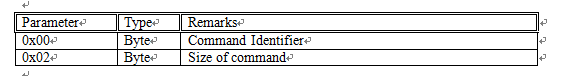所有命令都必须收到肯定或否定的答复。 否则，表明通信中断。 本节将列出常见或预期的答复。
否定确认（p。18）
无论命令如何，负状态值0x12始终可以作为答复。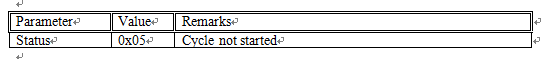Positive Acknowledge (see page 19)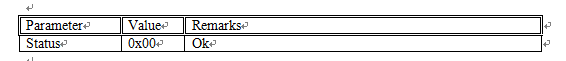Notes
任何其他可能相关的符号。
Description
该命令允许设备将消息保存到单元数据库中。
Format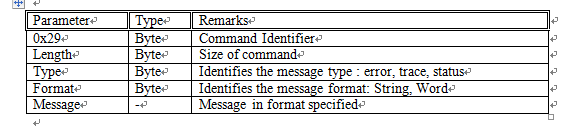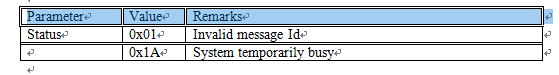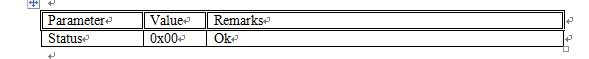Notes
Message Type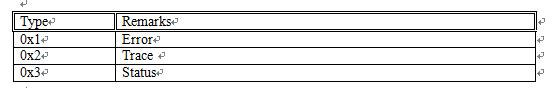Message Format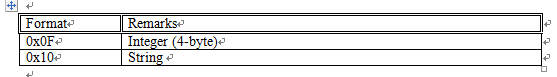Description
该命令在到达指定位置时由机器人发送。 Perceptron控制器使用它来验证是否应在预设的编码器值下进行测量。
展开全文机器学习
• 感知机实现与门(and)， 与非门(Nand)...class perceptron: @classmethod def AND(cls,x1, x2): w1, w2, b = 0.5, 0.5, -0.7 s = w1*x1+w2*x2+b return 1 if s>0 else 0 @classmethod def NAND(cls, x1, x2):
感知机实现与门(and)， 与非门(Nand)， 或门(or)， 异或门(xor)
原理python 实现
class perceptron:
@classmethod
def AND(cls,x1, x2):
w1, w2, b = 0.5, 0.5, -0.7
s = w1*x1+w2*x2+b
return 1 if s>0 else 0

@classmethod
def NAND(cls, x1, x2):
w1, w2, b = -0.5, -0.5, 0.7
s = w1*x1+w2*x2+b
return 1 if s>0 else 0

@classmethod
def OR(cls, x1, x2):
w1, w2, b = 0.5, 0.5, -0.3
s = w1*x1+w2*x2+b
return 1 if s>0 else 0

@classmethod
def XOR(cls, x1, x2):
return perceptron.AND(perceptron.NAND(x1,x2), perceptron.OR(x1, x2))

if __name__ == '__main__':
print("and")
print("0    0   {0}".format(perceptron.AND(0,0)))
print("0    1   {0}".format(perceptron.AND(0,1)))
print("1    0   {0}".format(perceptron.AND(1,0)))
print("1    1   {0}".format(perceptron.AND(1,1)))
print("nand")
print("0    0   {0}".format(perceptron.NAND(0,0)))
print("0    1   {0}".format(perceptron.NAND(0,1)))
print("1    0   {0}".format(perceptron.NAND(1,0)))
print("1    1   {0}".format(perceptron.NAND(1,1)))
print("or")
print("0    0   {0}".format(perceptron.OR(0,0)))
print("0    1   {0}".format(perceptron.OR(0,1)))
print("1    0   {0}".format(perceptron.OR(1,0)))
print("1    1   {0}".format(perceptron.OR(1,1)))
print("xor")
print("0    0   {0}".format(perceptron.XOR(0,0)))
print("0    1   {0}".format(perceptron.XOR(0,1)))
print("1    0   {0}".format(perceptron.XOR(1,0)))
print("1    1   {0}".format(perceptron.XOR(1,1)))

运行结果
and
0    0   0
0    1   0
1    0   0
1    1   1
nand
0    0   1
0    1   1
1    0   1
1    1   0
or
0    0   0
0    1   1
1    0   1
1    1   1
xor
0    0   0
0    1   1
1    0   1
1    1   0

参考
《深度学习入门：基于Python的理论与实现》
展开全文python 机器学习 深度学习 人工智能
•Python
• 使用Java构建的Perceptron模型。 导入库： const perceptron = require('./perceptron') 初始化模型（x =输入，y =目标）： let x = [[1, 1, 1], [0, 0, 0], [1, 0, 1]] let y = [1, 0, 1] let p = new ...JavaScript
• 感知器Java Java中的Perceptron神经网络示例Java
• Este programa apenas treina um perceptron（neurônio人工）para reconhecer umamatrículade 7dígitos。 以简单的神经元形式命名为todas，以自然语言形式命名为todas。 对等竞争者 gcc perceptron.c -o感知器
•Java
• python-perceptron 我不是一个聪明的名字命名者。 感知器是一种可以对线性可分离数据进行分类的算法。 它是最早的机器学习算法之一，其历史可以追溯到1950年代。 在我正在观看的它用作早期示例，因此我在Python中...
• matlab开发-Perceptron。感知器学习过程演示
• Deep Learning perceptron.pdf
• DL之perceptron：利用perceptron感知机对股票实现预测 目录 输出结果 实现代码 输出结果 更新…… 实现代码 import numpy as np import operator import os # create a dataset which ...
DL之perceptron：利用perceptron感知机对股票实现预测

目录
输出结果
实现代码

输出结果
更新……

实现代码
import numpy as np
import operator
import os

# create a dataset which contains 3 samples with 2 classes
def createDataSet():
# create a matrix: each row as a sample
group = np.array([[20,2], [50,1], [10,3],[60,0.5]])
labels = [1, -1, 1,-1] # four samples and two classes
return group, labels

#classify using perceptron
def perceptronClassify(trainGroup,trainLabels):
global w, b
isFind = False  #the flag of find the best w and b
numSamples = trainGroup.shape    #计算矩阵的行数
mLenth = trainGroup.shape    #计算矩阵的列数
w = *mLenth  #初始化w
b = 0   #初始化b
while(not isFind):  #定义迭代计算w和b的循环
for i in range(numSamples):
if cal(trainGroup[i],trainLabels[i]) <= 0: #计算损失函数，y（wx+b）<=0时更新参数
print (w,b)
update(trainGroup[i],trainLabels[i]) #更新计算w和b
break    #end for loop
elif i == numSamples-1:
print (w,b)
isFind = True   #end while loop

def cal(row,trainLabel): #定义损失函数
global w, b
res = 0
for i in range(len(row)):
res += row[i] * w[i]
res += b
res *= trainLabel
return res
def update(row,trainLabel): #学习率为1的更新计算
global w, b
for i in range(len(row)):
w[i] += trainLabel * row[i]
b += trainLabel

g,l =createDataSet()        #生成数据集
perceptronClassify(g,l)     #训练分类器


展开全文• Perceptron Approach from tkinter import * import numpy as np # 样本空间1 def pattern_one(event): global w1 x1, y1 = (event.x - 5), (event.y - 5) x2, y2 = (event.x + 5), (event.y + 5) w.c...
Perceptron Approach
from tkinter import *
import numpy as np

# 样本空间1
def pattern_one(event):
global w1
x1, y1 = (event.x - 5), (event.y - 5)
x2, y2 = (event.x + 5), (event.y + 5)
w.create_oval(x1, y1, x2, y2, fill='red')  # 实时获取的坐标作为参数
w1 = np.concatenate((w1, [[event.x, event.y, 1]]), axis=0)

# 样本空间2
def pattern_two(event):
global w2
x1, y1 = (event.x - 5), (event.y - 5)
x2, y2 = (event.x + 5), (event.y + 5)
w.create_oval(x1, y1, x2, y2, fill='green')  # 实时获取的坐标作为参数
w2 = np.concatenate((w2, [[-event.x, -event.y, -1]]), axis=0)

# 感知器算法
def judge():
global w1
global w2
W = np.random.random((1, 3))*10
X = np.concatenate((w1, w2), axis=0)
while True:
nc = 0
for x in X:
if np.dot(np.array([x]), W.T) <= 0:
W += np.array([x])
continue
nc += 1
if nc == np.shape(X):
x1 = 20
y1 = -(W[0, 2] + W[0, 0]*20)/W[0, 1]
x2 = 380
y2 = -(W[0, 2] + W[0, 0]*380)/W[0, 1]
print(y1, y2, W)
w.create_line(x1, y1, x2, y2, fill='white')
break

def change():
w.bind('<Button-1>', pattern_two)

def restart_program():
global w1
global w2
for item in w.find_all():
w.delete(item)
w.bind('<Button-1>', pattern_one)
w1 = np.empty((0, 3))
w2 = np.empty((0, 3))

if __name__ == '__main__':
# 初始化样本空间
w1 = np.empty((0, 3))
w2 = np.empty((0, 3))

root = Tk()
# 建立画布
w = Canvas(root, bg='blue', height=400, width=400)
w.pack()
# 绑定左键点击事件
w.bind('<Button-1>', pattern_one)
# 按钮部分
button = Frame(root).pack(side=BOTTOM)
Button(button, text='Change', command=change, width=18).pack(side=LEFT)
Button(button, text='Run', command=judge, width=18).pack(side=LEFT)
Button(button, text='Reset', command=restart_program, width=18).pack(side=RIGHT)
mainloop()

展开全文模式识别 算法
• Perceptron is a simple linear classifier.感知机
• Perceptron simple en Python paraclasificaciónde flores iris con scikitlearn 重要事项 Aunque el perceptron puede ser usado paraclasificación，tiene un问题和unc unc nunca收敛，没有完美的儿子可分离的...
• 感知器 可以直接运行以下三个脚本： standard_perceptron 投票的感知器 average_perceptronPython
• 一、题目：使用自己写的感知机Perceptron实现对鸢尾花数据的分类。 　数据来源： from sklearn.datasets import load_iris dataSet = load_iris() 二、注意事项： 　1）该数据中一共有三类，而感知机是个二...
• 计算机智能工程 作者： Sean Alvarenga Pedro Tanaka 要运行测试，请使用以下参数创建一个名为perceptron.properties的文件： threshold= learningRate= minWeight= maxWeight=Java
• //perceptron.h #ifndef PERCEPTRON_H__ #define PERCEPTRON_H__#include #include #include #include <string>class perceptron { public: float *weights; //权重 float b神经网络Python
•Python
• perceptron_THB.py -从头感知classifcation算法和感知学习算法的Implentation。 该文件进行测试和培训。 THB_data.py-使用泰国硬币的规格创建一个人工数据集。 数据 specs = { "1 satang": { "diameter": 15, # mm...Python...